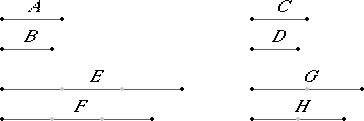# Proposition 16

If four magnitudes are proportional, then they are also proportional alternately.

Let A, B, C, and D be four proportional magnitudes, so that A is to B as C is to D.

I say that they are also so alternately, that is A is to C as B is to D.

V.Def.12Take equimultiples E and F of A and B, and take other, arbitrary, equimultiples G and H of C and D.

V.15

Then, since E is the same multiple of A that F is of B, and parts have the same ratio as their equimultiples, therefore A is to B as E is to F.

V.11

But A is to B as C is to D, therefore C is to D also as E is to F.

V.15

Again, since G and H are equimultiples of C and D, therefore C is to D as G is to H.

V.11

But C is to D as E is to F, therefore as E is to F also as G is to H.

V.14

But, if four magnitudes are proportional, and the first is greater than the third, then the second is also greater than the fourth; if equal, equal; and if less, less.

Therefore, if E is in excess of G, F is also in excess of H; if equal, equal; and if less, less.

V.Def.5

Now E and F are equimultiples of A and B, and G and H other, arbitrary, equimultiples of C and D, therefore A is to C as B is to D.

Therefore, if four magnitudes are proportional, then they are also proportional alternately.

Q.E.D.

## Guide

The statement says that a : b = c : d implies a : c = b : d. The proof goes as follows. Let m and n be numbers. Since both a : b = na : nb and c : d = mc : md, therefore na : nb = mc : md. By V.14, that implies

na >=< mc implies nb >=< md

so by the definition of proportion V.Def.5, a : c = b : d. Q.E.D.

#### No mixed ratios

The four magnitudes A, B, C, and D need to be of the same kind. If A and B are of a different kind than C and D, then the alternate ratios A : C and B : D would be “mixed.” The Greek geometers did not accept mixed ratios, but modern physicists and engineers routinely use them, as do we all since such a common measurement as velocity is made out of a ratio of a distance to a time.

This proposition requires using V.Def.4 as an axiom of comparability.

#### Use of this proposition

This proposition is used in V.19 and a couple others in Book V, and frequently in Books VI, X, XI, and XII. Occasionally it is used when the magnitudes need not be all of the same kind, as it ought not.